### Maloney Home Page  |  Electrothermal Actuation

#### Overview

The idea is simple: fabricate a beam connected to two anchors. When a voltage is applied to the beam, the flow of current causes it to heat up, expand, and deflect: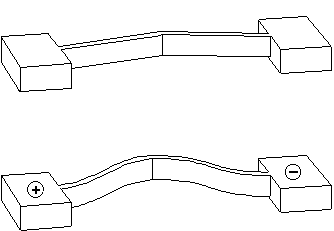The center of the beam is offset slightly to control the direction of deflection. The beam deflects far more than it would if it were simply cantilevered from a single anchor. The amplification factor can be calculated by using concepts from solid mechanics.

We designed and fabricated high-aspect-ratio thermal actuators from single-crystal silicon using deep reactive ion etching (DRIE). These images show the actuators at work: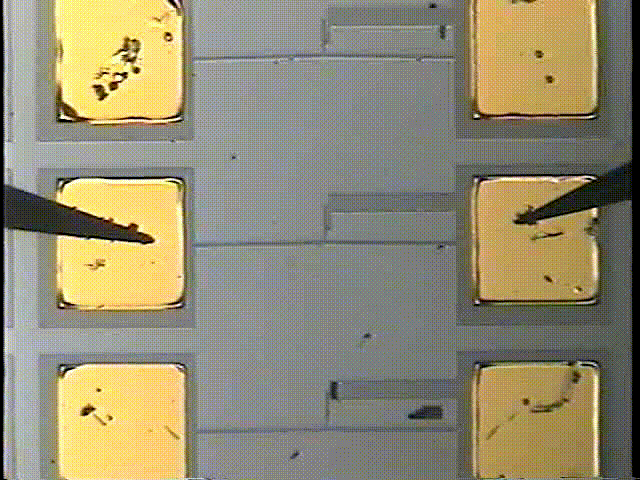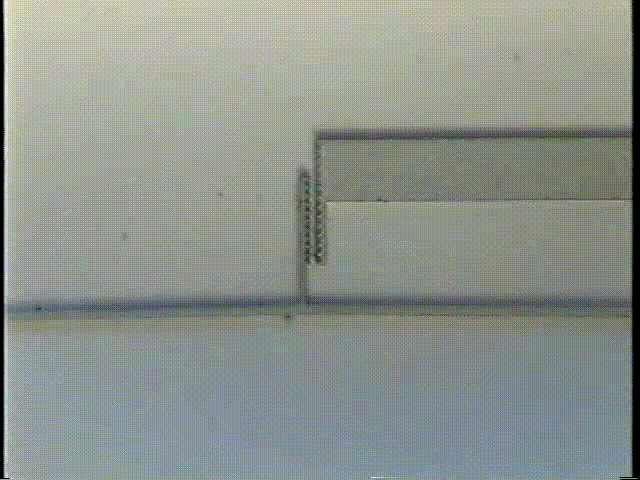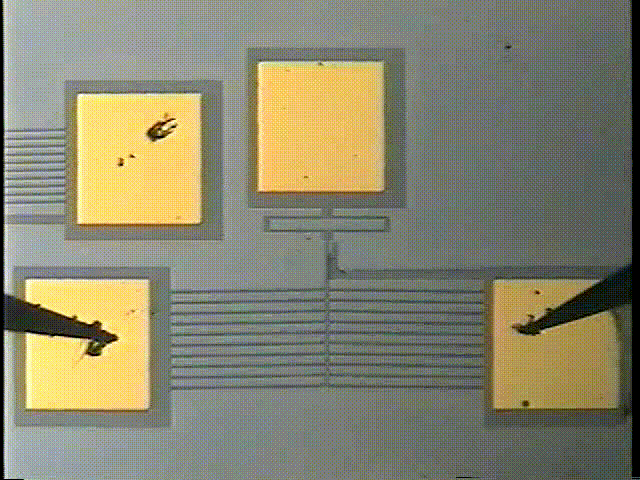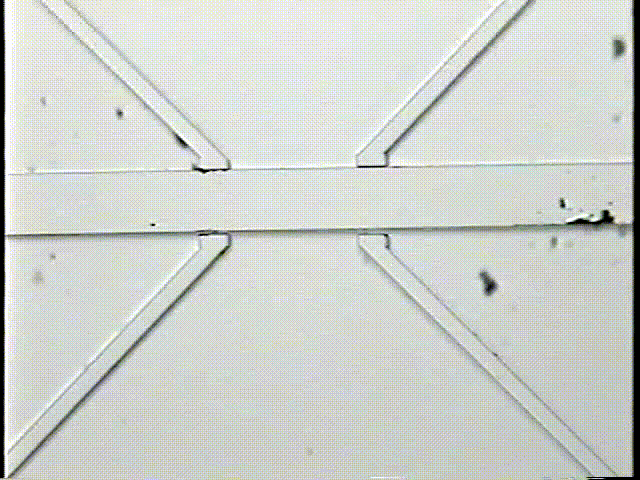(Animation created by using thermomechanical models in ANSYS 5.5; videos recorded from a CCD camera mounted on a probe station.) We go from the operational concept to a single 1 mm beam to arrays of beams, then to linear motors containing sliders nudged along by arrays.

The actuators are fabricated from silicon-on-insulator (SOI) wafers using metallization, DRIE, and HF etching. The HF etch is timed to release the beams without completely undercutting the large anchors. Gold pads on the anchors provide electrical connectivity. Vernier gauges are attached to the actuators to permit deflections to be measured.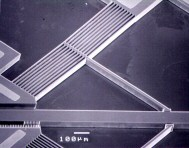High-aspect-ratio actuators fabricated with DRIE can generate significantly higher forces than surface micromachined polysilicon thermal actuators. Additionally, problems such as stiction and out-of-plane bowing are avoided. We have demonstrated deflections exceeding 25 µm and forces exceeding 2.5 mN for a current input of 20 mA.We have focused on V-beam actuators for motor applications because these actuators produce translational motion only, as opposed to the coupled translational and rotational motion produced by U-beam actuators. When fabricated in parallel arrays and connected by a center yoke, as shown in the video above, the resultant force scales up with the number of actuators.

In the linear motor, actuators are arranged in clamping and positioning arrays. The motor works by advancing a slider through frictional contact. By alternately positioning and clamping the slider, a very large range of motion is available. The clamping ability allows the motor to interact with compliant mechanisms and maintain a specified position. This capability makes this type of motor an excellent choice for integration with 3DMEMS devices.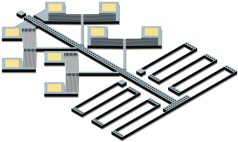The efficiency of these thermal motors is extremely low, approximately 0.0000001. This low efficiency limits the use of microactuators in low-power applications such as self-sufficient robotics. However, the actuators are useful in stationary applications. For example, the nanotechnology company Zyvex uses thermal actuators in a nanomanipulation system.

#### Modeling

A key part of this work is the estimate of the time constant of these thermal actuators.

Because the length of a typical actuator beam is considerable larger than height or width, a 1-D differential element is used to model the temperature distribution in the silicon. This element, with height h, width w, and thickness dx, is illustrated below, where the current flux J represents the current per unit area through the element.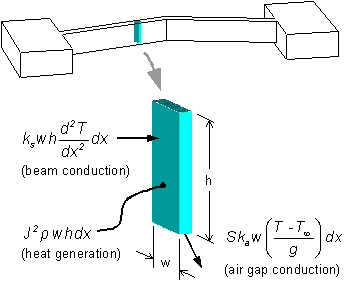In this diagram, the current flux J represents the current per unit area through the element. The material properties shown are the resistivity ρ, the silicon thermal conductivity ks, and the air thermal conductivity ka.

It has been shown that, in the case of a very small gap under a suspended beam, the effects of convection and radiation are negligible and conduction through air to the substrate dominates. However, conduction from the sides of the beam through the surrounding air to the substrate is not negligible and must be accounted for by the shape conduction factor S. This parameter is typically found via finite element analysis.

Because of the relatively large thickness of the substrate (525 µm), the large 600 µm × 600 µm anchors, and the high thermal conductivity of single-crystal silicon, the substrate and anchor temperatures are assumed to remain at ambient temperature. Finite element analysis of the complete geometry has shown this assumption to be reasonable. The thermal conductivity of air and the resistivity of silicon are assumed to be independent of temperature. Also, the thermal conductivity and thermal expansion coefficient of silicon are initially assumed to be independent of temperature to make the heat equation tractable.

By using the differential element above and dividing by the element volume, the time-independent heat equation can be written as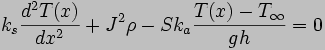The transient response of the V-beam thermal actuators can be predicted by examining the time-dependent form of this equation: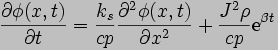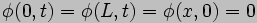where the values of the specific heat c and density p of silicon are assumed to be independent of temperature, and the following variable substitutions have been made: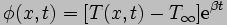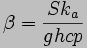Applying the finite Fourier transform to the time-dependent equation results in the following nonhomogeneous differential equation:where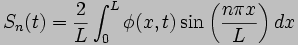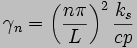This equation has the solution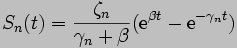to which the inverse finite Fourier transform can be applied: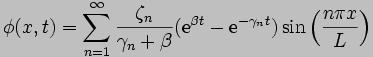The general solution is therefore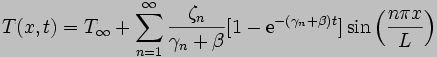implying a first-mode time constant of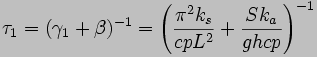which offers a useful way of comparing heat loss contributions from beam conduction and air conduction. The first term represents heat loss through the beam to the anchors; the second term represents heat loss through the air gap to the substrate. By using typical values, a typical V-beam actuator with a length L of 1000 µm, a width w of 10 µm, and a height h of 50 µm is predicted to have a first-mode time constant τ1 of approximately 0.8 ms. The corresponding cut-off frequency is approximately 200 Hz.

Transient experiments were performed on the V-beam actuators with a laser Doppler vibrometer. These tests were performed by mounting a chip vertically and focusing the laser on the side of an actuator beam. The normalized frequency response is shown below. As expected, the full range of motion is maintained at low frequencies. As the frequency increases past 100 Hz, response drops 20 dB per decade because the actuator cannot complete each heating/cooling cycle fast enough to keep up with the signal. By fitting a curve to the frequency response, the time constant of this actuator is estimated at 1.6 ms, as shown as the solid line below.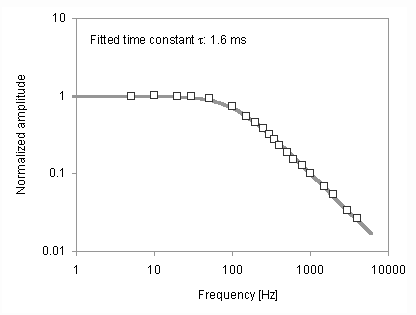It would seem that the transient model is off by a factor of two. In fact, the earlier calculation was performed with the room temperature values of silicon thermal conductivity and specific heat. However, we know from resistance measurements and from visual observation of glowing silicon that portions of the actuators exceed 500°C during operation. What happens if high-temperature values are used? If we take the material properties at around 500°C, the calculated time constant is 1.8 ms. So the experimental results are actually bounded by estimates using temperatures of 25°C and 500°C. For better accuracy, we would need to model the beam by multiple elements, each with its own temperature and material properties.

#### Impact

This work was published here in 2004. Since then, our modeling and fabrication work has been cited by over 100 papers investigating electrothermal actuation and its applications and by the books Modeling MEMS and NEMS by Pelesko and Bernstein (CRC Press, 2002), RF MEMS: Theory, Design, and Technology by Rebeiz (Wiley 2003), Micromanufacturing and Nanotechnology by Mahalik (Springer, 2005) and MEMS Linear and Nonlinear Statics and Dynamics by Younis (Springer, 2011).

 © Copyright John M. Maloney